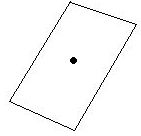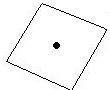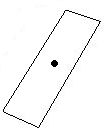I am currently working on a mapping program in which polygons, rectangles, circles, etc... are rotated, moved, and resized. Recently, I used the rotation conversion formula to rotate the items n degrees/radians by using the point and the center point of the shape.
Currently, I am working on resizing the shape (length and/or width) while still trying to maintain the integrity of the shape. I basically want to decrease or increase the y value by 1 in order to resize the length and I want to decrease or increase the x value by 1 in order to resize the width.
The corresponding x and y values either increase by 1, stay the same, or decrease by 1.
Is it possible to use just the point and center of the shape in order to calculate this resizing?
What can I use to accomplish this task?

Thank you,

Anthony

Anthony,

It is not clear what you want. If you add the same number to each x coordinate, and another number to each y-coordinate you simply translate the original figure -- it retains its original size, shape, and orientation.

If you want to add different numbers to different y-coordinates, you distort the figure in an unpredictable way.

If you want to change the size of the figure you should multiply the x coordinate by a number b and the y coordinate by a number c. This stretches the figure out and increases (or decreases) the area by the factor bc. To maintain the shape of the figure, just let b = c.

Chris

To clarify my previous question...I am creating a computer program that will resize any polygon shape in a picture box, which has all positive X,Y coordinates beginning with (0,0).
When I resize the shape by multiplying the X,Y coordinate by the same number (ie., X * 1.25 and Y * 1.25), the shape enlarges but the center point has moved, thus moving the shape.
I need to enlarge or shrink the length or width of the shape while maintaining the same center point for the shape. For example:Original ShapeReduced LengthReduced Width

The two factors that I have to work with are the center point of the shape

xCenter = (the highest X value of the shape + the lowest X value of the shape) / 2,
yCenter = (the highest Y value of the shape + the lowest Y value of the shape) / 2

and each (X,Y) coordinate for each vertex of the shape.
I have recently been looking at ellipses, but I am currently stuck on determining the minor axis for the ellipse from the data I currently have.
Would the equation for an ellipse work for this, or is there another approach that I am not seeing?

Thank you,

Anthony,

Suppose that the center has coordinates (c,d), s is the scaling factor in the x direction and t is the scaling factor in the y direction. For any point P with coordinates (x,y) you want to transform P by

x is transformed to s(x - c) + c
y is transformed to t(y - d) + d

Chris